Mirrored from Sudopedia, the Free Sudoku Reference Guide

# Hypersudoku

## Hypersudoku

Hypersudoku, Windoku, NRC-Sudoku, or Four-square Sudoku is one of the Sudoku Variations with additional constraints. This format was first introduced in the Dutch newspaper NRC Handelsblad by Peter Ritmeester and the first playable online version by Chris McCusker.

## How to Play Hypersudoku

This game requires no mathematics skills. It is purely a logic game with the only prerequisite to be able to count to nine.

Hypersudoku Rules
The rules for playing hypersudoku are very simple.
1. All rows must have all the numbers from 1 - 9 in them (none can be repeated). There are 9 rows in the game.

2. All columns must have all the numbers from 1 - 9 in them (none can be repeated). There are 9 columns in the game.

3. All 3x3 squares must have all the numbers from 1 - 9 in them (none can be repeated). There are 13 squares in the game. There are the 9 underlaying 3x3 squares (divided by the dark blue lines) and the 4 overlaying (shown in light blue in the following diagrams).

## Hypersudoku Logic

### New Game

Hypersudoku is a game of logic. The following is a brief explanation of the logic necessary to develop your strategies for the game of hypersudoku.
As you will see in the following diagrams there are many starting points for the game in figure 1. This is a fairly typical layout for an easy level hypersudoku game. These are only a few of the many starting points in this particular game.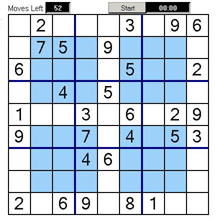Figure 1

### Example1

Looking at the center square we can see that column 4 has a 9 at the bottom(circled in red). This means that a nine can not appear again in this column.

Column 5 also has a 9 in it. This time up in the top middle square.

This leaves column 6 as the only option for a 9 to appear in the center square. As 6 and 4 take up 2 of the 3 squares available, there is only one square left. This is the one with the blue tick in it. This square will contain the 9.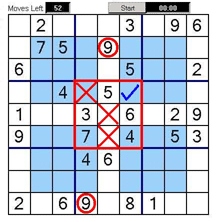Figure 2

### Example 2

In figure 3 if we look at the top right square, we can see that rows 2 and 3 already have a 5 (circled in red) in them.
This leaves the square with the blue tick as the only possibility for a 5 in this square.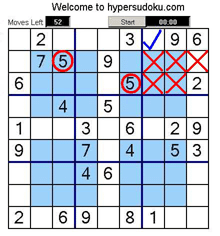Figure 3

### Example 3

Again the same starting game, but this time looking at the middle right square. Rows 4 and 6 contain a 4 already, leaving only row 3.
As the other 2 squares already contain numbers, the square with the blue tick is the only square that can contain a 4.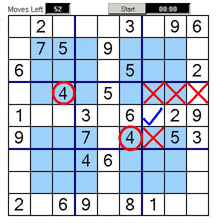Figure 4

### Example 4

In figure 5 we can see that the top row and third row already have a 6 in them. The sixth column also has a 6 in it.
This leaves the square with the blue tick as the only square that could contain a 6 in this block.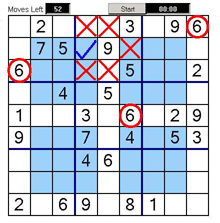Figure 5

### Example 5

If we look at the bottom center square we can see that columns 5 and 6 both contain a 5 (circled in red).
This leaves only one square in this block that can contain a 5.(square with blue tick)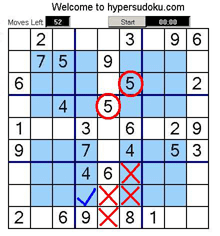Figure 6

### Example 6

In figure 7 we see that column 1 and 2 have a 2 in them already. Also note that row 5 has a 2 in it. (circled in red)
Again this only leaves the square with the blue tick in it as the only possible square in this block that could contain a 2.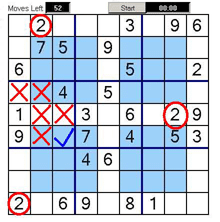Figure 7

### Example 7

If we look at the top right blue square, it has to have a nine in it. The top corner square (in red) that overlaps the blue square already has a nine in it (circled in red). The middle top square that also overlaps the blue square has a nine already in it (circled in red). The middle right-hand square also has a nine in it which only leaves the square with the blue tick as the only possible square for nine to go in in the top right blue square.

As you can see the logic of the overlapping squares is quite helpful for solving these puzzles.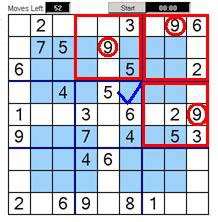Figure 8

### Example 8

In this example if we look at the bottom left blue square, it has to have a six in it. The bottom corner square (in red) that overlaps the blue square already has a six in it (circled in red). This six is also the only six that can exist in this column so that eliminates the top middle blue square (marked with red cross) .The middle bottom square that also overlaps the blue square has a six already in it (circled in red). This only leaves the square with the blue tick as the only possible square for six to go in in the bottom right blue square.

As you can see the logic of the overlapping squares is quite helpful for solving these puzzles.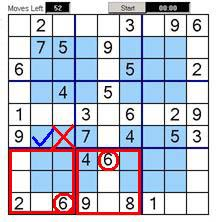Figure 6

There are many possible first moves to the start of the hypersudoku game. These are certainly by no means the only starting moves for this particular game.

## Hidden Constraints

Although there are only 4 additional constraints mentioned by the publisher, the position of these 4 windows indirectly creates 5 additional constraints. These are shown in the following picture.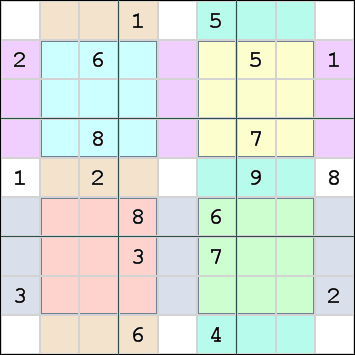## Solving Techniques

The solving techniques for a Windoku are similar to those of regular Sudoku. The extra constraints are placed in such a way that there are many more intersections to deal with. Each additional constraint can contain subsets and strong links which could be used in coloring techniques.

There are fewer Unique Rectangles in the grid, because not all 468 possible unique rectangles for a standard Sudoku are located in exactly 2 additional houses.

## Minimum Number of Givens

Valid Windokus with 11 givens exist. However, it is not known whether this is the minimum number of givens. See this page for examples for such Windokus.